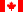Canadian Version Return to Calculator : Help - Contents

### N=Noe-(0.693t/T½)

Use this equation to find out how much of a radioactive material is left after a given period of time.

To use the equation:
The information required by the equation is the initial activity (No), the time elapsed since the initial activity was measured (t), and the half-life of the isotope (T½).

1. Select the isotope from the list at the top of the page; this provides the equation with the isotope's half-life. If your isotope is not on the list, you can still use the equation if you manually enter the half-life in the Half-life textbox at the top of the page. You can get half-life values here.
2. Next enter the initial activity, No. It is not necessary to worry about units here, just keep in mind that the units for the final activity will be the same as for the initial activity.
3. Enter the time elapsed, t, since the initial activity was measured.
4. Select the correct time unit. If the time unit is not available, you will have to convert the time elapsed to a unit that is available.
5. Click the Calculate button and the final activity is displayed.

An example:
You purchase 9.25 µCi of P-32, but it sits for 9 days before you get a chance to use it. How much of the original 9.25 µCi is left?

1. Select Phosphorus 32 from the isotope list. 14.26 and d show up in the Half-life and Half-life time unit textboxes.
2. Enter 9.25 in the Initial activity (No) textbox.
3. Enter 9 in the Decay time (t) textbox and select the d radio button.
4. Click the Calculate button and the answer 5.97 is received in the Final activity (N) textbox.